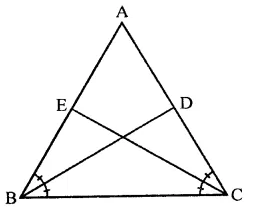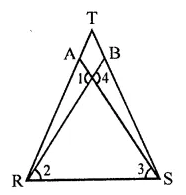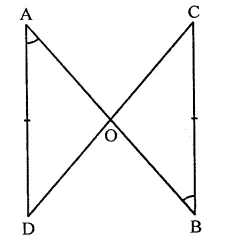# RD Sharma Class 9 Solutions Chapter 12 Heron’s Formula Ex 12.2

## RD Sharma Class 9 Solutions Chapter 12 Heron’s Formula Ex 12.2

These Solutions are part of RD Sharma Class 9 Solutions. Here we have given RD Sharma Class 9 Solutions Chapter 12 Heron’s Formula Ex 12.2

Other Exercises

Question 1.
BD and CE are bisectors of ∠B and ∠C of an isosceles ∠ABC with AB = AC. Prove that BD = CE.
Solution:
Given : In ∆ABC, AB = AC
BD and CE are the bisectors of ∠B and ∠C respectively
To prove : BD = CE
Proof: In ∆ABC, AB = AC
∴ ∠B = ∠C (Angles opposite to equal sides)
∴ $$\frac { 1 }{ 2 }$$ ∠B = $$\frac { 1 }{ 2 }$$ ∠C∠DBC = ∠ECB
Now, in ∆DBC and ∆EBC,
BC = BC (Common)
∠C = ∠B (Equal angles)
∠DBC = ∠ECB (Proved)
∴ ∆DBC ≅ ∆EBC (ASA axiom)
∴ BD = CE

Question 2.
In the figure, it is given that RT = TS, ∠1 = 2∠2 and ∠4 = 2∠3. Prove that: ∆RBT = ∆SAT.Solution:
Given : In the figure, RT = TS
∠1 = 2∠2 and ∠4 = 2∠3
To prove : ∆RBT ≅ ∆SAT
Proof : ∵ ∠1 = ∠4 (Vertically opposite angles)
But ∠1 = 2∠2 and 4 = 2∠3
∴ 2∠2 = 2∠3 ⇒ ∠2 = ∠3
∵ RT = ST (Given)
∴∠R = ∠S (Angles opposite to equal sides)
∴ ∠R – ∠2 = ∠S – ∠3
⇒ ∠TRB = ∠AST
Now in ∆RBT and ∆SAT
∠TRB = ∠SAT (prove)
RT = ST (Given)
∠T = ∠T (Common)
∴ ∆RBT ≅ ∆SAT (SAS axiom)

Question 3.
Two lines AB and CD intersect at O such that BC is equal and parallel to AD. Prove that the lines AB and CD bisect at O.
Solution:
Given : Two lines AB and CD intersect each other at O such that AD = BC and AD $$\parallel$$
BCTo prove : AB and CD bisect each other
i. e. AO = OB and CO = OD
Proof: In ∆AOD and ∆BOC,